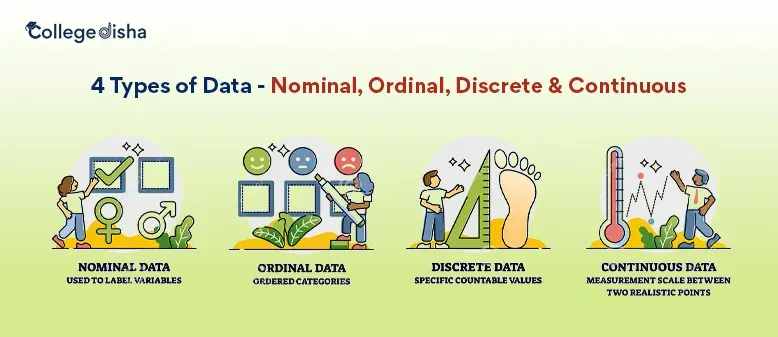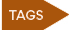Update on 03 Nov, 22

# 4 Types of Data - Nominal, Ordinal, Discrete & Continuous

4 Types of Data - Nominal, Ordinal, Discrete & Continuous: Data Science is most crucial for businesses to thrive in today’s world. Data is an asset for every organization as it helps to understand the market and reduces wastage of money and saves time. Every business whether small, medium or large use raw or structured data to derive insights and design new strategies that will ultimately optimize ROI.

A large quantity of data is produced on a daily basis and it is very important to process the different types of data in order to gather valuable information that will generate more leads in business. It is, therefore, necessary to store, handle and analyze large sets of data to prevent any error and derive the best results out of it.

In the process of storing data, it is also essential to understand the type of statistical analysis that will best fit the data type to derive meaningful insights. This requires a good understanding of the data types and data categories.

Data can be divided into two major classes- Qualitative Data and Quantitative Data which are the most used categories of Data. These can be further subdivided into Nominal, Ordinal, Discrete and Continuous.

## Types of Data:

Qualitative Data (Also known as Categorical Data)

This type of data is nonnumerical in nature. Since this data type is sorted by categories and not by numbers, it is also known as categorical data. This type of data describes the qualities and information about the individual.

Qualitative data falls in the types of data that helps to understand the mindset of the customer and answer questions like “how much” or "how often”. Qualitative data is unique to an individual and consists of physical traits, images, texts, symbols, audio recordings, descriptions, case studies, etc.

Examples of Qualitative Data:

• Hair colors
• Temperature
• Holiday destinations
• Opinion (Agree or disagree)
• Weight of a person
• Mode of transportation

Qualitative data can be further subdivided into 2 types- Nominal and Ordinal Data.

## ➜ Nominal Data:

Nominal data is a qualitative type of data that does not have any numerical value and is used for naming variables. The term Nominal comes from the Latin word “Nomen” meaning name. The Nominal Data belongs to the types of data that are unique and cannot be measured. Nominal data does not have any common elements and cannot be altered even if the order of the data is changed.

Some examples of Nominal Data are the following:

• Personality types (introvert, extrovert)
• Gender (Male, Female)
• Employment status (Employed, Unemployed, Retired)
• Mode of transport (bus, car, train, etc)
• Marital status (Married, Unmarried, Single)
• Hair Color (Black, White, Blonde, Red)
• Languages spoke (English, Spanish, French, etc)

From the above examples, we see that Nominal Data is mainly descriptive and there is no definite ordering or hierarchy in the datasets. This type of data is unique and it is not possible to calculate the mean of such data types.

## ➜ Ordinal Data:

Ordinal data belongs to the Qualitative types of Data that have a definite order by their position on a scale. This is a categorical type of data where the variables can be placed in a hierarchy. However, there is no clear distance between the hierarchies or levels. Ordinal Data shows only the sequence and can be numbered but cannot be calculated.

Therefore, Ordinal Data is considered in-between Qualitative and Quantitative Data.

Some examples of Ordinal Data are the following:

• Level of agreement (Agree, Disagree, strongly agree, Neither agree nor disagree)
• Grades in the exam (A, B, C, D)
• Time of the day (Morning, Afternoon, Night)
• Income level (Lower, Middle, Upper)
• Purchasing experience (Satisfactory, Neutral, Somewhat satisfactory)

Since the Ordinal data are not numerical in nature, it is not possible to calculate these types of Data directly. In fact, numbers can be assigned to the Ordinal Data but they cannot be calculated. Therefore label encoding can be applied to Ordinal Data to fit the data into Machine Learning models and derive insights from it.

### Difference Between Nominal and Ordinal Data:

 Nominal Data Ordinal Data Data does not have any order Data has some distinct ordering Data is qualitative or categorical Data is between qualitative and quantitative Data cannot be arranged in sequence Data can be arranged in sequence One data type cannot be compared with another Data types can be compared through ranking

➜ Quantitative Data (Also known as Numerical Data)

Quantitative Data consists of unique datasets that have a numerical value and define a quantity, amount, or range. These data can be mathematically derived by using calculations. Quantitative types of data can be counted and measured and are suitable for statistical analysis.

There can be an infinite number of numerical values of the Quantitative Data that can be measured. Quantitative Data involves answering questions like “how much”, “how many” or “how often”.

Examples of quantitative data:

• Number of weeks (in a year)
• Number of students (in a class)
• Age (in months or years)
• Room temperature
• Scores in the exam
• Weight of a person (in kg)
• Quantitative Data can be further subdivided into 2 types- Discrete and Continuous

### ➜ Discrete Data:

These are the types of data that can take only certain values that are finite values and cannot be subdivided. Discrete data can only be counted in whole numbers or integers and cannot be broken down into decimals. Discrete data is mainly represented by tally charts, frequency tables, or bar graphs.

The best way to represent Discrete datasets is by using bar graphs as the values can be shown using horizontal or Vertical bars.

Some examples of Discrete Data are the following:

• Number of students in a Class
• The favorite sport of a number of people
• Number of questions answered correctly during an exam
• Number of languages spoken by an individual
• Shoe sizes of a group of people

The above examples indicate that Discrete Data can only be counted in whole numbers and cannot be subdivided or measured. Discrete data types can also be Ordinal or Nominal depending upon the rank that they can fit into.

The types of Discrete data that fit into categories that have no order are Nominal Discrete Data whereas Discrete Data that fit into categories with order is Ordinal Discrete data.

### ➜ Continuous Data

Continuous Data are the type of Data that can take any value that falls within a range. Continuous Data can change over time. Continuous Data can be measured on an infinite scale and can take any value on the scale that includes a fractional value.

It is possible to calculate the average and Standard Deviation of Discrete Data. Continuous Data types can be represented by Histograms, line graphs, or skews.

Some examples of Continuous Data are the following:

• Speed of cars
• Height of children
• Speed of a vehicle
• Time is taken in a race
• Daily wind speed
• The temperature of a deep fridge

The above examples indicate that the variables in each set of data can be stretched to decimal points. Continuous Data when plotted on a graph will represent a distinct line. Continuous Data can have different values at different time intervals.

### Difference Between Discrete and Continuous Data:

 Discrete Data Continuous Data Finite sets of Data Infinite sets of Data Data consists of whole numbers or integers Data consists of fractions or decimals Data cannot be divided into subdivisions Data can be divided into subdivisions Data has spaces between the values Data is in the form of a continuous sequence

### Importance of Data Classification:

Data classification is necessary to track and segregate data so that it can be easily searched and analyzed. By classifying data it is possible to protect and maintain the confidentiality of sensitive data. In order to safeguard Data, it is necessary to know the different types of data and how they can be protected.

Classified data helps organizations to meet regulatory compliances and overcome legal hurdles whenever necessary. It also helps in the retrieval of lost data and mitigates the risk factors associated with leakage of sensitive data.

Data classification is mandatory to bridge security gaps in the data and helps to monitor the access of valuable data both internally and externally. It helps in reducing the risk of data loss and sets policies regarding the sharing of valuable data in an organization.

### Statistical Tests on Classified Data:

After the classification of different types of data into different categories, it is essential to perform statistical tests on the datasets to understand patterns and derive insights. Given below is the list of tests conducted on classified datasets.

ANOVA Test: Used to check the impact of one or more factors on two or more groups of variables. ANOVA or the analysis of variance is applicable only to qualitative datasets.

Chi-Square Test: This test is used to compare two categorical variables. This test is used to determine whether sample data fit a population. A Chi-square test will compare two categorical variables and see whether they differ from each other.

Regression Analysis: Regression analysis is used to determine the relationship between a dependent variable and two or more independent variables to understand their future relation. Regression analysis involves linear regression, multiple linear regression, and nonlinear regression.

Summary:

It is very important to know the data types before working on the data. Classification of data types is necessary for the visualization and analysis of data. Every organization, big or small relies on data-driven decisions to enhance productivity and acquire valuable information.

Therefore it is important to know the kinds of data, how it can be categorized and what type of statistical tests can be performed on it to get the best output. Data classification is a key component for businesses to understand the type of data they possess and ways to safeguard the data.

Classified data can be easily stored, managed, and protected to reduce wastage and save time.